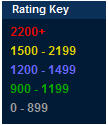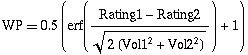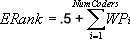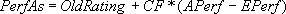# Algorithm Ratings

The following statistics are kept about each coder:

• Rating
• Volatility
• Number of times previously rated

Before competing, new members ratings are provisional. After a challenge, the algorithm below is applied to the competitors. First, the ratings of members who have previously competed are calculated, with new members' performances not considered. Second, new members are given a rating based on their performance relative to everyone in the challenge.A coder's handle is color-coded depending on their rating within the Arena.

## How ratings are calculated

New ratings are calculated as follows: After each challenge, each coder who attended the challenge is re-rated according to the following algorithm. For Algorithm Challenges, keep in mind that only coders who shared the same problem set are rated against one another. For Marathon Matches, a coder is considered to have attended the event is they make a submission of any kind (example or full). Only registering for the event will not cause the coder to be rated. The average rating of everyone in the challenge is calculated:Where NumCoders is the number of coders in the challenge and Rating is the rating without the volatility of the coder in the challenge before the challenge.

The challenge factor is calculated:Where Volatility is the volatility of the coder in the challenge before the challenge.

Win Probability Estimation Algorithm:Where Rating1 & Vol1 are the rating and volatility of the coder being compared to, and Rating2 & Vol2 are the rating and volatility of the coder whose win probability is being calculated. Erf is the "error function".

The probability of the coder getting a higher score than another coder in the challenge (WPi for i from 1 to NumCoders) is estimated.

The expected rank of the coder is calculated:The expected performance of the coder is calculated:Where Φ is the inverse of the CDF of the standard normal distribution.

The actual performance of each coder is calculated:Where ARank is the actual rank of the coder in the challenge based on score (1 for first place, NumCoders forlast). If the coder tied with another coder, the rank is the average of the positions covered by the tied coders.

The performed as rating of the coder is calculated:The weight of the challenge for the coder is calculated:Where TimesPlayed is the number of times the coder has been rated before. To stabilize the higher rated members, the Weight of members whose rating is between 2000 and 2500 is decreased 10% and the Weight of members whose rating is over 2500 is decreased 20%.

A cap is calculated:The new volatility of the coder is calculated:The new rating of the coder is calculated:If |NewRating - Rating| > Cap the NewRating is adjusted so it is at most Cap different than Rating.